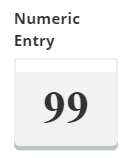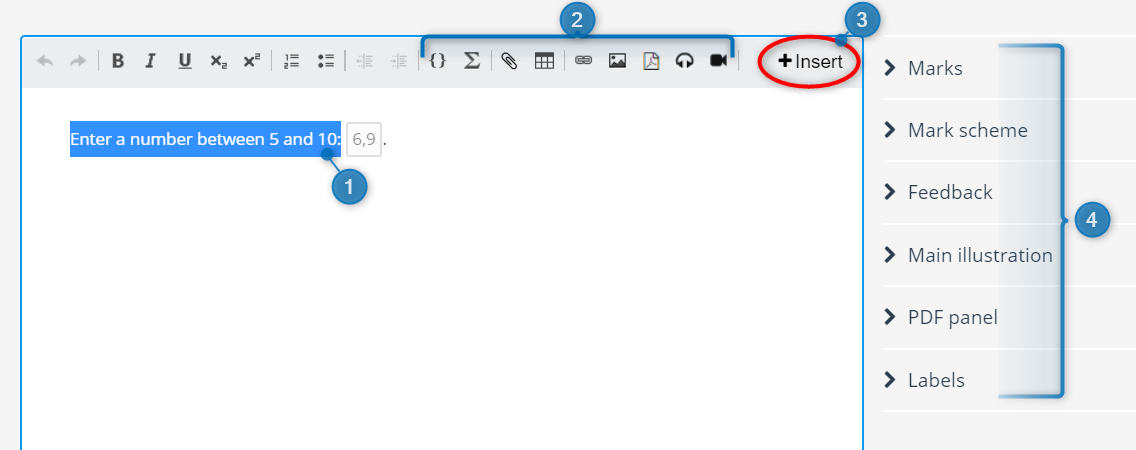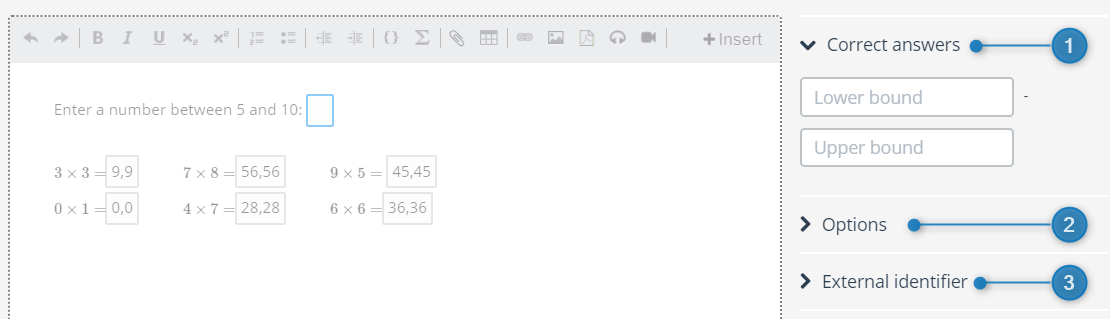# Question type - Numeric EntryAutomatically marked question. The question is answered by typing a numeric value.

Numeric Entry is a question type where the candidates can answer the question by writing only a number or number value. If a candidate uses lettes, they will receive an error message.

In the question's edit mode, you can do the following:1. Replace text with desired question text.

2. Add math (LaTex), files, tables, links, PDF, images and / or audio clips to the question text.

3. Insert multiple interaction elements by pressing +Insert:4. Set general options for the question type. Read more about general options for questions

## Specific options

Clicking on the interaction element gives you the specific options for the question type.1. Correct answers: In this option you can define the correct number range for the answer. It you want the answer to be a single number, just enter the same number in the lower and upper bound.

2. Options: Select the number of decimals and the length of the interaction field.

• Excepted number of decimals: This option will either cut the candidate's response (if the candidate has entered more decimals than requested), or it will add decimals up to the maximum requested (if the candidate has entered fewer than requested).
• Expected length (characters): Select the length of the selected interaction element. The length is chosen by entering the number of expected characters to be answered by the candidate.
• Restrict number of characters to input width: Sets the maximum allowable candidate input to the same length as the input width.
Note: Make sure that this is greater or equal to the number of characters in the correct answer. If it is shorter, the candidate will not be able to answer correctly.

3. External identifier: For information on external identifier, read general options for questions